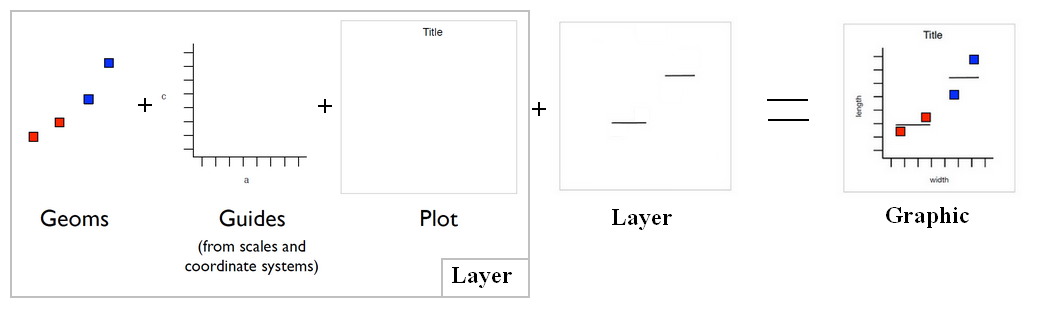# Ggplot - Point (geom_point, geom_jitter) (Scatterplot)

A geom point to create Scatterplot

## Management

### Size

````geom_point(aes(size = qsec))`
```

### Jitter to reduce overplotting

current/geom_jitter.html is a shortcut for geom_point(position = “jitter”)

Points are jittered to reduce overplotting.

````geom_jitter(width = 2, height = 5, alpha = 0.5)`
```

## Example

### Categorical Data Ordered By Continuous Value

``````f = factor(c('six','one', 'two','three','four','five'))
x = c(4,1,5,2,10,3)

# We re[[order|order]] the factor by x
f <- reorder(f,x,sum)
#  where:
#     * f is a factor of length n,
#     * x is a vector of length n (It can also be negative if the sort must be descendant)
#     * the third parameter is a function (sum, median) that the lapply function supports.

ggplot()+geom_point(aes(x=x,y=f))```
```

Recommended PagesGGplot - Layer (data + mapping + geom + stat + position)

Layer = data: The data frame (in a variable or a function, if null inherited) + mapping: Mapping data to geom properties (ie aesthetics) + geom : The geometric object to use display the data +...Ggplot - Geom (geometric objects) - type of plot

Geom is a short for geometric objects that describe the type of plot produced. point for point (to create a scatterplot) line for a line ... The geom_... functions are shortcuts for a layer set...Ggplot - Order

Order in Ggplot A factor is plotted ordered by its level, you can reorder it with the reorder function. By default, the order is alphabetical, The plot is not ordered by x but by the alphabet...Ggplot - Point (geom_point, geom_jitter) (Scatterplot)

A geom point to create Scatterplot geom_jitter is a shortcut for geom_point(position = “jitter”) Points are jittered to reduce overplotting.